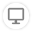# MathUtils

Stable version 3.0.9 (Compatible with OutSystems 11)
Other versions available for 10 and Older
Published on 15 July 2021 by
4.9
(12 ratings)# MathUtils

Documentation
3.0.9

For using this component just download it from the forge, install the component on your environment and update the dependencies on the desired module. All the functions should be available on the logic tab for you to use them in standard outsystems flows.

When you have all the server actions available on your module, you can use them on screens, you should define the input variables and output variables, adjusting the rest of the flow according to your needs. In the image below is an example of a standard flow using one of the actions available on this component:Here is a list of all the functions available:

Math_Acos

Returns the arccosine (in radians) of a cosine.

Math_AreaCircle

Returns the area of a circle given a radius.

Math_AreaEllipse

Returns the area of an ellipse given the two semi axes (major and minor).

Math_AreaRect

Returns an area of a rectangle given the width and height.

Math_AreaSector

Returns an area of a circle's sector given his radius and angle (radians).

Math_AreaSquare

Returns the area of a square given its side.

Math_AreaTriangle

Returns an area of a triangle using the triangle's three sides (Heron's formula).

Math_Asin

Returns the arcsine (in radians) of a number.

Math_Atan

Returns the arctangent (in radians) of a number.

Math_Atan2

Returns the angle in the plane (in radians) between the positive x-axis and the ray from (0,0) to the point (x,y).

Math_BigMul

Returns the product of two 32-bit numbers.

Math_Ceiling

Rounds a number up to the next largest integer.

Math_Cos

Returns the cosine of the specified angle.

Math_Cosh

Returns the hyperbolic cosine of a number.

Math_DivRem

Calculates the quotient between two numbers and the remainder.

Math_E

Returns the natural logarithmic base, specified by the constant, e.

Math_Exp

Returns e^x, where x is the argument, and e is Euler's number.

Math_Floor

Returns the largest integer less than or equal to a given number.

Math_IEEERemainer

Returns the remainder of a division operation.

Math_Log

Returns the natural logarithm (base e) of a number

Math_Log10

Returns the base 10 logarithm of a number.

Math_Log2

Returns the base 2 logarithm of a number.

Math_LogBase

Calculates a number given the base and the logarithm.

Math_Pi

Returns the value of the mathematical constant Pi.

Math_Random

Generates a random number between zero and the selecter number.

Math_RandomRange

Generates a random number in a range defined by a min and max value.

Math_RoundAwayFromZero

Rounds a number with the desired number of decimals.

Math_RoundToEven

Rounds a number using the round-to-even method.

Math_Sign

Returns either a positive or negative +/- 1, indicating the sign of a number passed into the argument.

Math_Sin

Returns a numeric value between -1 and 1, which represents the sine of the angle given in radians.

Math_Sinh

Returns the hyperbolic sine of a number.

Math_Sqrt

Returns the square-root of a given number.

Math_Truncate

Returns the designed number truncated.

Math_Tan

Returns the tangent of a number.

Math_Tanh

Returns the hyperbolic tangent of a number.

Math_CelsiusToFahrenheit

Converts a number from Celsius to Fahrenheit.

Math_CentimetersToInches

Converts a number from Centimeters to Inches.

Math_CoordinateBearing

Calculates the bearing, in degrees, between two sets of coordinates on Earth.

Math_CoordinateDistance

Calculates the distance between two sets of coordinates on Earth, in meters.

Converts a number from Degrees to Radians.

Math_FahrenheitToCelsius

Converts a number from Fahrenheit to Celsius.

Math_InchesToCentimeters

Converts a number from Inches to Centimeters.

Math_KilosgramsToPounds

Converts Kilograms into Pounds.

Math_KilometersToMiles

Converts Kilometers into Miles.

Math_MilesToKilometers

Converts Miles into Kilometers.

Math_NormalizeDeg

Normalizes an angle in Degrees.